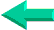This is an excerpt from Pierre Leclerc (www.excel-vba.com)

The 2 Most Important

Excel Functions AND
Formulas

SUMPRODUCT

AND

INDEX/MATCH

## Annex 8: Excel Spreadsheets  Mathematical Functions (59)

There are 59 functions in the "Mathematical" category.

 Functions What it Does SUM Adds its arguments SUMPRODUCT The most powerful and useful function in Excel ROUND Rounds a number to a specified number of digits ROUNDUP Rounds a number up, away from zero SUBTOTAL Returns a subtotal of a filtered list or database) TRUNC Truncates a number to an integer INT Rounds a number down to the nearest integer) ABS Returns the absolute value of a number MOD Returns the remainder from division POWER Returns the result of a number raised to a power SQRT Returns a positive square root EVEN Rounds a number up to the nearest even integer EXP Returns e raised to the power of a given number FLOOR Rounds a number down, toward zero ROUNDDOWN Rounds a number down, toward zero GCD Returns the greatest common divisor LCM Returns the least common multiple ODD Rounds a number up to the nearest odd integer MROUND Returns a number rounded to the desired multiple PRODUCT Multiplies its arguments QUOTIENT Returns the integer portion of a division RAND Returns a random number between 0 and 1 RANDBETWEEN Returns a random number between the numbers you specify ROMAN Converts an arabic numeral to roman, as text SIGN Returns the sign of a number CEILING Rounds a number to the nearest integer or to the nearest multiple of significance COMBIN Returns the number of combinations for a given number of objects ACOS Returns the arccosine of a number ACOSH Returns the inverse hyperbolic cosine of a number ASIN Returns the arcsine of a number ASINH Returns the inverse hyperbolic sine of a number ATAN Returns the arctangent of a number ATAN2 Returns the arctangent from x- and y-coordinates ATANH Returns the inverse hyperbolic tangent of a number COS Returns the cosine of a number COSH Returns the hyperbolic cosine of a number DEGREES Converts radians to degrees FACT Returns the factorial of a number LN Returns the natural logarithm of a number LOG Returns the logarithm of a number to a specified base LOG10 Returns the base-10 logarithm of a number MDETERM Returns the matrix determinant of an array MINVERSE Returns the matrix inverse of an array MMULT Returns the matrix product of two arrays MULTINOMIA Returns the multinomial of a set of numbers PI Returns the value of pi RADIANS Converts degrees to radians SERIESSUM Returns the sum of a power series based on the formula SIN Returns the sine of the given angle SINH Returns the hyperbolic sine of a number SQRTPI Returns the square root of (number * pi) SUMIF Adds the cells specified by a given criteria SUMSQ Returns the sum of the squares of the arguments SUMX2MY2 Returns the sum of the difference of squares of corresponding values in two arrays SUMX2PY2 Returns the sum of the sum of squares of corresponding values in two arrays SUMXMY2 Returns the sum of squares of differences of corresponding values in two arrays TAN Returns the tangent of a number TANH Returns the hyperbolic tangent of a number FACTDOUBLE Returns the double factorial of a number In Excel 2007 and Up SUMIFS Adds the cells specified by one or many given criteria (SUMPRODUCT does better)

If you are using a version of Excel earlier than 2007 you need to activate the "Excel Analysis Toolpack" to make some of the functions above available

SUMPRODUCT  ¦  INDEX/MATCH  ¦  SUBTOTAL  ¦  ISERROR  ¦  HLOOKUP  ¦  LOOKUP  ¦  VLOOKUP
AVERAGEIF  ¦  AVERAGEIFS ¦  COUNTIF  ¦  COUNTIFS  ¦  SUMIF  ¦  SUMIFS  ¦  IFERRORBack home